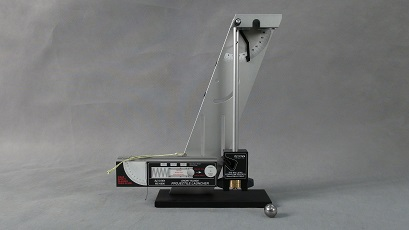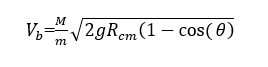# Mb-7 Ballistic Pendulum

## Aim

To demonstrate that momentum is transferred from one body to another, during a collision.  Can be used to calculate the velocity of a projectile just before the moment of the collision.

## Apparatus

• Ballistic Pendulum
• Large Ball Bearing
• PlungerBallistic Pendulum.

## Description

Load the ball bearing into the launcher using a plunger. Set onto the maximum range. Zero the angle indicator by hand. Launch the ball bearing by pulling the string on the launcher. The ball bearing will be fired into the catcher at the end of the pendulum. The pendulum will be propelled, and the angle indicator will be moved to end of the range of the pendulum. The speed of the ball bearing can be calculated from the angle of range of pendulum, mass of pendulum, and mass of the ball bearing. This demonstrates the initial method that speed of a bullet was measured.

The equation gives an approximate speed of the ball bearing• Where M is mass of pendulum
• m is mass of ball bearing
• R length of pendulum in cm
• θ is the angle the pendulum reaches